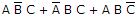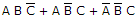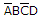# Digital Electronics - Boolean Algebra and Logic Simplification

### Exercise :: Boolean Algebra and Logic Simplification - General Questions

11.

An AND gate with schematic "bubbles" on its inputs performs the same function as a(n)________ gate.

 A. NOT B. OR C. NOR D. NAND

Answer: Option C

Explanation:

No answer description available for this question. Let us discuss.

12.

For the SOP expression, how many 1s are in the truth table's output column?

 A. 1 B. 2 C. 3 D. 5

Answer: Option C

Explanation:

No answer description available for this question. Let us discuss.

13.

A truth table for the SOP expressionhas how many input combinations?

 A. 1 B. 2 C. 4 D. 8

Answer: Option D

Explanation:

No answer description available for this question. Let us discuss.

14.

How many gates would be required to implement the following Boolean expression before simplification? XY + X(X + Z) + Y(X + Z)

 A. 1 B. 2 C. 4 D. 5

Answer: Option D

Explanation:

No answer description available for this question. Let us discuss.

15.

Determine the values of A, B, C, and D that make the product termequal to 1.

 A. A = 0, B = 1, C = 0, D = 1 B. A = 0, B = 0, C = 0, D = 1 C. A = 1, B = 1, C = 1, D = 1 D. A = 0, B = 0, C = 1, D = 0

Answer: Option A

Explanation:

No answer description available for this question. Let us discuss.

#### Current Affairs 2021

Interview Questions and Answers